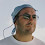## Tuesday 8 March 2016

### Excel Tip: Using Index to calculate a cumulative sum (e.g. a Year to Date total)

Let me start with an apology. My current workload has meant that my posts have been a lot less frequent in recent weeks. This may continue for a while, but I will still be aiming to post at least once a month during this busy spell.

In this post I want to show you how to do something I have shown before using OFFSET, but this time using the more efficient INDEX.

Let's look at the example of a 12 month budget spreadsheet with the monthly sales figures in cells B9 to M9 (month 1 in B9, Month 2 in C9, etc.). And let's say that the current month number is entered in cell B6.

Using OFFSET we could return the current month's sales using:

=OFFSET(\$B9,0,\$B\$6-1)

and the cumulative sales using:

=SUM(OFFSET(\$B9,0,0,1,\$B\$6))

See this earlier post, if you don't understand why.

The problem with OFFSET though, is that it is what is known as a "volatile" function. This means that it always has to be recalculated when any cell in the spreadsheet changes - as it does not specify a range that it is dependent on, therefore does not know whether a change might affect its result.

INDEX however looks at a defined range, making it significantly more efficient, and in its simplest form can be used to replace both of these examples of OFFSET.

For a range the width of a single cell (as in our example), INDEX only needs two arguments to return the current month's sales:

=INDEX(\$B9:\$M9,\$B\$6)

This returns the value of the cell in position B6 in the range B9:M9.

If \$B\$6 is 3, this will return the value in cell D9, being the third cell in the range B9:M9.

To do the cumulative calculation, we can use the same INDEX function to return the end of  a SUM range, while fixing the start:

=SUM(\$B9:INDEX(\$B9:\$M9,\$B\$6))

Again, if B6 contains 3, then this returns the sum of the range B9:D9.

In both cases, these will only recalculate if a cell in the range B9:M9 is edited. In a complex spreadsheet with many calculations, this can make a huge difference to calculation times.

If you enjoyed this post, go to the top of the blog, where you can subscribe for regular updates and get two freebies "The 5 Excel features that you NEED to know" and "30 Chants for Better Charts".

1.good to know, now I'll just go and redesign all my spreadsheets…

2.Before recoding I would check the likely savings. In some cases they may not be as much as one might expect. For example, if the range from which the value is being selected is a table column containing equations dependent on other columns in the table. In this case changing any value in the table will cause the index function to recalculate.
The offset function has the advantage of conferring protection from inserted and deleted rows for example when doing a running total.
Geoff W

3.Nice example of use of Index. Will hopefully remember it. Thanks.

4.I also wouldn't go recoding all formulae in your workbooks where you need to return the MTD and YTD values for line items in your P&L. If the range holding the MTD values are not moving (i.e. the table is pretty well fixed in location barring insertion/deletion of rows), then direct referencing of those cells in a simple SUM formulae to return the YTD is still the best way. Where I'd use Glen's technique is to extract values for a selected line item (where this selection changes from time to time i.e. in a more dynamic situation.)

1.The issue this is intended to address though, is that no matter how fixed the range is, it will still change each month, summing 4 months in month 4 and 5 in month 5 - for example. A simple SUM formula would need to be edited each month, creating more work and more potential for error.

5.Nice solution for a variable sum. Thnx!

6.